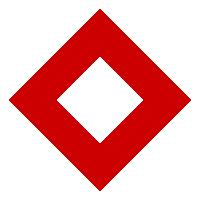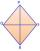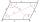# Diamond

Side length of diamond is 35 cm and the length of the diagonal is 56 cm. Calculate the height and length of the second diagonal.

v =  16.8 cm
u =  42 cm

### Step-by-step explanation:Did you find an error or inaccuracy? Feel free to write us. Thank you!Tips to related online calculators
Do you want to convert length units?
Pythagorean theorem is the base for the right triangle calculator.

#### You need to know the following knowledge to solve this word math problem:

We encourage you to watch this tutorial video on this math problem:

## Related math problems and questions:

• Diamond sideThe diagonals of the diamond are 18 cm and 14 cm long. Calculate the length of the diamond side.
• Diamond diagonalsCalculate the diamonds' diagonals lengths if the diamond area is 156 cm square, and the side length is 13 cm.
• Diamond diagonalsCalculate the diamond's diagonal lengths if its content is 156 cm2 and the side length is 13 cm.
• Rhombus diagonalsIn the rhombus ABCD are given the sizes of diagonals e = 24 cm; f = 10 cm. Calculate the side length of the diamond and the size of the angles, calculate the content of the diamond
• Rhombus IVCalculate the length of the diagonals of the rhombus, whose lengths are in the ratio 1: 2 and a rhombus side is 35 cm.
• Diamond diagonalsFind the diamond diagonal's lengths if the area is 156 cm2 and side is 13 cm long.
• Rhombus and inscribed circleIt is given a rhombus with side a = 6 cm and the radius of the inscribed circle r = 2 cm. Calculate the length of its two diagonals.
• DiamondCalculate the length of the two diagonals of the diamond if: a = 13 cm v = 12 cm
• DiamondDetermine the side of diamond if its content is S = 353 cm2 and one diagonal u2 = 45 cm.
• The diamondThe diamond has sides of 35 cm and the diagonals are in a ratio of 1: 2. Calculate the lengths of the diagonals.
• RhombusCalculate the perimeter and area of ​​a rhombus whose diagonals are 39 cm and 51 cm long.
• Diagonals in diamons/rhombusRhombus ABCD has side length AB = 4 cm and a length of one diagonal of 6.4 cm. Calculate the length of the other diagonal.
• RhombusIt is given a rhombus of side length a = 19 cm. Touchpoints of inscribed circle divided his sides into sections a1 = 5 cm and a2 = 14 cm. Calculate the radius r of the circle and the length of the diagonals of the rhombus.
• RhombusOne angle of a rhombus is 136°, and the shorter diagonal is 8 cm long. Find the length of the longer diagonal and the side of the rhombus.
• Rhombus and diagonalsThe rhombus area is 150 cm2, and the ratio of the diagonals is 3:4. Calculate the length of its height.
• RhombusThe rhombus has diagonal lengths of 4.2cm and 3.4cm. Calculate the length of the sides of the rhombus and its height
• The diamondThe diamond has an area S = 120 cm2, the ratio of the length of its diagonals is e: f = 5: 12. Find the lengths of the side and the height of this diamond.